Between cities A and B is route 13 km long of stúpanie average 7‰. Calculate the height difference of cities A and B.

h =  91 m

### Step-by-step explanation:Did you find an error or inaccuracy? Feel free to write us. Thank you!Tips to related online calculators
Do you want to convert length units?

#### You need to know the following knowledge to solve this word math problem:

We encourage you to watch this tutorial video on this math problem:

## Related math problems and questions:

• Height differenceWhat height difference is overcome if we pass a road 1 km long with a pitch21 per mille?
• RailwayRailway line had on 5.8 km segment climb 9 permille. How many meters track ascent?
• Slope of trackCalculate the average slope (in permille and even in degrees) of the rail tracks between Prievidza (309 m AMSL) and Nitrianske Pravno (354 m AMSL), if the track is 11 km long.
• ClimbFor horizontal distance 4.2 km road rise by 6.3 m. Calculate the road pitch in ‰ (permille, parts per thousand).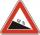The road sign informs the gradient is 10.9%. Calculate the angle at which the average decreases.
• Mountain railway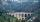Height difference between points A, B of railway line is 38.5 meters, their horizontal distance is 3.5 km. Determine average climb in permille up the track.
• Climb in percentageThe height difference between points A and B is 475 m. Calculate the percentage of route climbing if the horizontal distance places A, B is 7.4 km.
• ClimbOn the road sign, which informs the climb is 8.7%. The car drive 5 km along this road. What is the height difference that the car went to?
• Two municipalities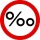The horizontal distance between municipalities is 39 km. Average sinking is 7 per mille. What is the difference in height between these municipalities?The average climb of the road is given by ratio 1:15. By what angle road average climb?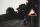A 5 km long road begins at an altitude 500 meters above sea level and ends at an altitude of 521 ASL. How many permille road rises?Railways climb 7.4 ‰. Calculate the height difference between two points on the railway distant 3539 meters.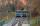Funicular on Petrin (Prague) was 408 meters long and overcomes the difference 106 meters in altitude. Calculate the angle of climb.Calculate how many permille river Dunaj average falls, if on section long 957 km flowing water from 1454 m AMSL to 101 m AMSL.The angle of a straight road is approximately 12 degrees. Determine the percentage of this road.There is a traffic sign for climbing on the road with an angle of 7%. Calculate at what angle the road rises (falls).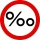What a climb in per mille of the hill long 4 km and the altitude difference is 6 meters?